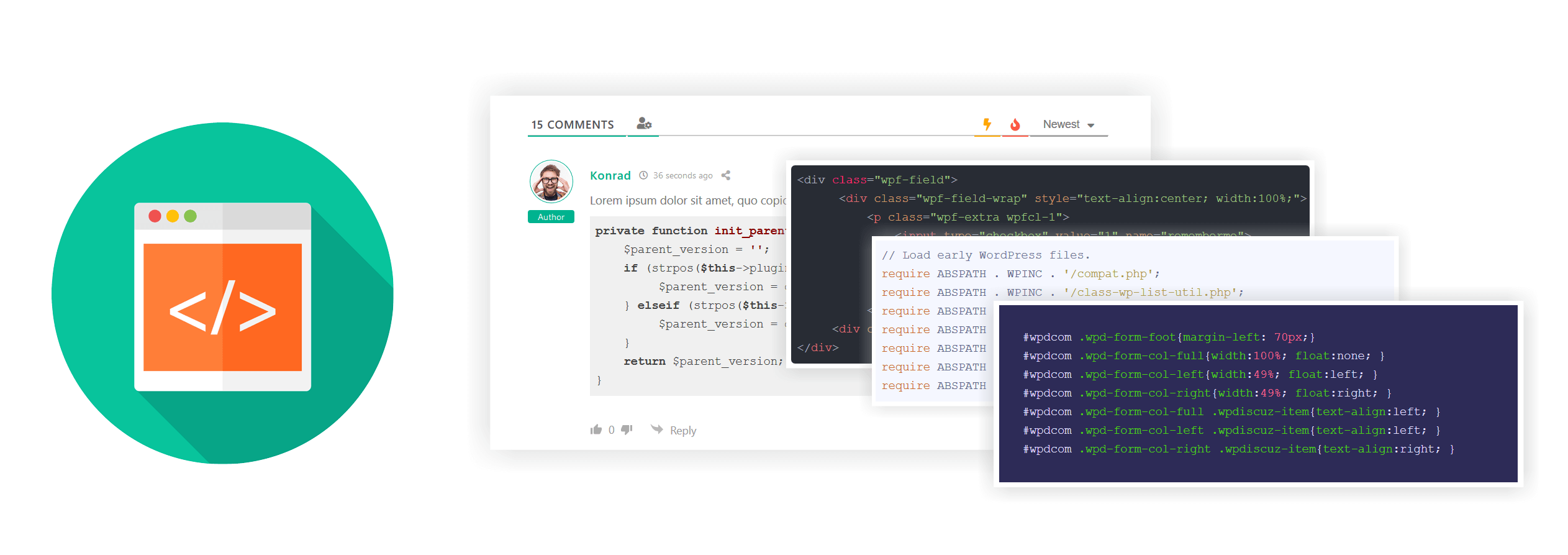# wpDiscuz – Syntax HighlighterwpDiscuz Syntax Highlighter addon displays formatted source code using the highlight.js JavaScript library in comment content. This addon supports almost all programming languages including Apache, Bash, C#, C++, CSS, HTML, XML, JSON, Java, JavaScript, Objective-C, PHP, Perl, Python, Ruby, SQL, Basic, Delphi, Erlang, Matlab, Prolog, ScalaScheme, Swift, TypeScript, VB.NET, Shell with dozens of different syntax highlighting styles. With this addons your commenters can insert different scripts and codes using the [</>] code toolbar button in comment editor. In the dashboard settings you can enable/disable certain programming language and change the highlighting style.

Article Rating
Subscribe
Notify ofInline FeedbacksRichard
4 months ago

PHP

```<?php
class Car {
function Car() {
\$this->model = "Tesla";
}
}

// create an object
\$Lightning = new Car();

// show object properties
echo \$Lightning->model;
?>
```Richard
4 months ago

Java Script

```// ES5 syntax
function Person() {
// we assign `this` to `self` so we can use it later
var self = this;
self.age = 0;

setInterval(function growUp() {
// `self` refers to the expected object
self.age++;
}, 1000);
}

// ES6 syntax
function Person(){
this.age = 0;

setInterval(() => {
// `this` now refers to the Person object, brilliant!
this.age++;
}, 1000);
}

var p = new Person();
```Ann
4 months ago
```<!DOCTYPE html>
<html>
<body>

<h2>JavaScript Strings</h2>

<p>Strings can be written with double or single quotes.</p>

<p id="demo"></p>

<script>
document.getElementById("demo").innerHTML = 'John Doe';
</script>

</body>
</html>
```
Last edited 4 months ago by AnnAnn
4 months ago
```body {
background-image: url("barn.jpg");
background-repeat: repeat-y;
}

.text1 {
font-size: 16px;
}
@media (min-width: 1200px) {
.text1 {
font-size: 20px;
}
}
```Ann
4 months ago
```//this is the single line comment

/** This is multiline comment,
compiler ignores any code inside comment blocks.
**/

//This is the namespace, part of the standard .NET Framework Class Library
using System;
// namespace defines the scope of related objects into packages
namespace Learning.CSharp
{
// name of the class, should be same as of .cs file
public class Program
{
//entry point method for console applications
public static void Main()
{
//print lines on console
Console.WriteLine("Hello, World!");
//Reads the next line of characters from the standard input stream.Most common use is to pause program execution before clearing the console.
}
}
}
```Joseph
4 months ago
```def get_numbers_stack(list)
stack  = [[0, []]]
output = []

until stack.empty?
index, taken = stack.pop

next output << taken if index == list.size

stack.unshift [index + 1, taken]
stack.unshift [index + 1, taken + [list[index]]]
end

output
end
```Joseph
4 months ago
```# Python Program to find the area of triangle

a = 5
b = 6
c = 7

# Uncomment below to take inputs from the user
# a = float(input('Enter first side: '))
# b = float(input('Enter second side: '))
# c = float(input('Enter third side: '))

# calculate the semi-perimeter
s = (a + b + c) / 2

# calculate the area
area = (s*(s-a)*(s-b)*(s-c)) ** 0.5
print('The area of the triangle is %0.2f' %area)
```Kelvin
3 months ago

Hello, i agreeKelvin
3 months ago

Ok, testinguLA
2 months ago

nONE

Last edited 2 months ago by uLAuLA
2 months ago
```ФУНКЦИЯ ОпрИзРСПоследнийОтветОтПользователя(Пользователь, ВидСообщения, МИД = НЕОПРЕДЕЛЕНО, ИсключитьКомандуИзПоиска = НЕОПРЕДЕЛЕНО)

Запрос = НОВЫЙ Запрос;
Запрос.УстановитьПараметр("Пользователь", Пользователь);

Запрос.Текст =
"ВЫБРАТЬ ПЕРВЫЕ 1
|	ИС.Период КАК Период,
|	ИС.message_id,
|	ИС.Команда,
|	ИС.ОбъектВзаимодействия
|ИЗ
|	РегистрСведений.tel_ИсторияСообщений КАК ИС
|ГДЕ
|	ИС.Пользователь = &amp;Пользователь
|	И ИС.ВидСообщения = &amp;ВидСообщения";
ЕСЛИ НЕ МИД = НЕОПРЕДЕЛЕНО ТОГДА
Запрос.УстановитьПараметр("МИД", МИД);
Запрос.Текст = СтрЗаменить(Запрос.Текст,
"	И ИС.ВидСообщения = &amp;ВидСообщения",
"	И ИС.ВидСообщения = &amp;ВидСообщения И ИС.message_id = &amp;МИД");
КОНЕЦЕСЛИ;
ЕСЛИ ВидСообщения = НЕОПРЕДЕЛЕНО ТОГДА
Запрос.Текст = СтрЗаменить(Запрос.Текст, "И ИС.ВидСообщения = &amp;ВидСообщения", "");
ИНАЧЕ
Запрос.УстановитьПараметр("ВидСообщения", ВидСообщения);
КОНЕЦЕСЛИ;
```Daniel
21 days ago

Get-PnPSitesDaniel
21 days ago
```Get-PnPSites
```Daniel
21 days ago
```UpdateContext({cvarUser : User})
```Test
16 days ago
```=IF(MOD(IF(MID(\$D\$2,1,1)*2>9,MID(\$D\$2,1,1)*2-9,MID(\$D\$2,1,1)*2)+MID(\$D\$2,2,1)+IF(MID(\$D\$2,3,1)*2>9,MID(\$D\$2,3,1)*2-9,MID(\$D\$2,3,1)*2)+MID(\$D\$2,4,1)+IF(MID(\$D\$2,5,1)*2>9,MID(\$D\$2,5,1)*2-9,MID(\$D\$2,5,1)*2)+MID(\$D\$2,6,1)+IF(MID(\$D\$2,7,1)*2>9,MID(\$D\$2,7,1)*2-9,MID(\$D\$2,7,1)*2)+MID(\$D\$2,8,1)+IF(MID(\$D\$2,9,1)*2>9,MID(\$D\$2,9,1)*2-9,MID(\$D\$2,9,1)*2)+MID(\$D\$2,10,1)+IF(MID(\$D\$2,11,1)*2>9,MID(\$D\$2,11,1)*2-9,MID(\$D\$2,11,1)*2)+MID(\$D\$2,12,1)+IF(MID(\$D\$2,13,1)*2>9,MID(\$D\$2,13,1)*2-9,MID(\$D\$2,13,1)*2)+MID(\$D\$2,14,1)+IF(MID(\$D\$2,15,1)*2>9,MID(\$D\$2,15,1)*2-9,MID(\$D\$2,15,1)*2)+MID(\$D\$2,16,1),10)=0,VLOOKUP(--LEFT(\$D\$2,6),A1:B100,2,0),"NOT OK")
```Dima
12 days ago

.wrapper {
display: flex;
flex-direction: column;
min-height: 100%;
}Dima
12 days ago
```.wrapper {
display: flex;
flex-direction: column;
min-height: 100%;
}
```